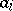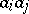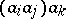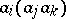The square multiplication table of an arbitrary finite groupoid. Heading the table is a list of symbols denoting all elements of the groupoid (in any order); these symbols (in the same order) are also listed in front of the first column. If the groupoid has an identity element, the latter is usually put first. If the symbol in the-th position of the prefacing column is, and that in the-th position of the heading row is, then the entry at the intersection of the-th row and the-th column is the symbol denoting the product. Cayley tables were first used by A. Cayley in 1854 for groups (cf. Group).
A Cayley table defines a quasi-group if and only if each row (column) contains each symbol exactly once. The Cayley table of a group must satisfy the following additional condition: The positions corresponding to the productsandcontain identical elements. Cayley tables which are symmetric about the principal diagonal represent commutative binary operations; in particular, this is the case for the Cayley table of an Abelian group.